# Physics Assignment Help With Work Done by Gravity

## Work Done by Gravity

Consider a block of mass m which slides down a smooth inclined plane of angle qas shown in figure. Let us assume the coordinate axes as shown in the figure, to specify the components of the two vectors - although the value of work will not depend on the orientation of the axes.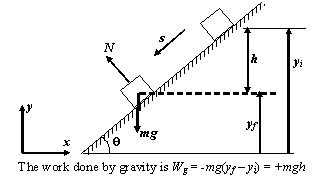Now, the force of gravity,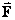g = -mg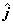and the displacement is given by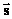= Dx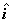+ Dy+ Dz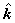The work done by gravity is
Wg =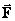g.= -mg.(Dx+ Dy+ Dz)

or Wg = -mgDy (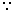.= 0,.= 1,.= 0)

Since Dy = yf - yi = -h
\ Wg = -mg(yf - yi) = +mgh

If the block moves in the upward direction, then the work done by gravity is negative and is given by
Wg = -mgh

### Assignment Help For Work Done by Gravity

assignmenthelp.net provides best Online Assignment Help service in Physics for all standards. Our Tutor provide their high quality and optimized Tutorial help to fulfill all kind of need of Students.